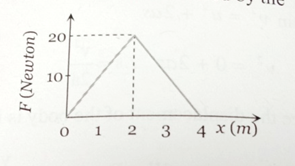# NEET Physics Mathematical Tools Questions Solved

The graph between the resistive force F acting on a body and the distance covered by the body is shown in the figure. The mass of the body is 25 kg and initial velocity is 2 m/s. When the distance covered by the body is 4 m, its kinetic energy would be    [This question is only for Dropper and XII batch]1. 50 J

2. 40 J

3. 20 J

4. 10 J